Munster tutorial
Date
March 11, 2015

This document describes the PLUMED tutorial held in Munster, March 2015. The aim of this tutorial is to learn how to use PLUMED to analyze molecular dynamics simulations on the fly, to analyze existing trajectories, and to perform enhanced sampling. Although the presented input files are correct, the users are invited to refer to the literature to understand how the parameters of enhanced sampling methods should be chosen in a real application.

Users are also encouraged to follow the links to the full PLUMED reference documentation and to wander around in the manual to discover the many available features and to do the other, more complete, tutorials. Here we are going to present only a very narrow selection of methods.

We here use PLUMED 2.1 syntax and we explicitly note if some syntax is expected to change in PLUMED 2.2, which will be released later in 2015. All the tests here are performed on a toy system, alanine dipeptide, simulated using the AMBER99SB force field. We provide both a setup that includes explicit water, which is more realistic but slower, and a setup in gas phase, which is much faster. Simulations are made using GROMACS 4.6.7, which is here assumed to be already patched with PLUMED and properly installed. However, these examples could be easily converted to other MD software.

All the gromacs input files and analsys scripts are provided in this tarball .

Users are expected to write PLUMED input files based on the instructions below.

# Alanine dipeptide: our toy model

In this tutorial we will play with alanine dipeptide (see Fig. munster-1-ala-fig). This rather simple molecule is useful to make benchmark that are around for data analysis and free energy methods. It is a nice example since it presents two metastable states separated by a high (free) energy barrier. Here metastable states are intended as states which have a relatively low free energy compared to adjacent conformations. It is conventional use to show the two states in terms of Ramachandran dihedral angles, which are denoted with $$\Phi$$ and $$\Psi$$ in Fig. munster-1-transition-fig .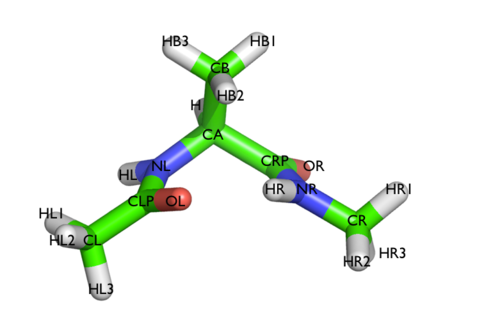The molecule of the day: alanine dipeptide.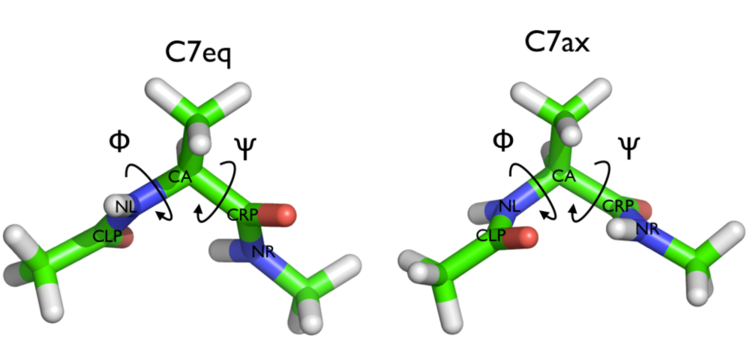Two metastable states of alanine dipeptide are characterized by their Ramachandran dihedral angles.

# Monitoring collective variables

The main goal of PLUMED is to compute collective variables, which are complex descriptors than can be used to analyze a conformational change or a chemical reaction. This can be done either on the fly, that is during molecular dynamics, or a posteriori, using PLUMED as a post-processing tool. In both cases one should create an input file with a specific PLUMED syntax. A sample input file is below:

# compute distance between atoms 1 and 10
d: DISTANCE ATOMS=1,10
# create a virtual atom in the center between atoms 20 and 30
center: CENTER ATOMS=20,30
# compute torsional angle between atoms 1,10,20 and center
phi: TORSION ATOMS=1,10,20,center
# compute some function of previously computed variables
d2: MATHEVAL ARG=phi FUNC=cos(x) PERIODIC=NO
# print both of them every 10 step
PRINT ARG=d,phi,d2 STRIDE=10


(see DISTANCE, CENTER, TORSION, MATHEVAL, and PRINT)

PLUMED works using kJ/nm/ps as energy/length/time units. This can be personalized using UNITS. Notice that variables should be given a name (in the example above, d, phi, and d2), which is then used to refer to these variables. Lists of atoms should be provided as comma separated numbers, with no space. Virtual atoms can be created and assigned a name for later use. You can find more information on the PLUMED syntax at Getting started page of the manual. The complete documentation for all the supported collective variables can be found at the Collective variables page.

## Analyze on the fly

Here we will run a plain MD on alanine dipeptide and compute two torsional angles on the fly. GROMACS needs a .tpr file, which is a binary file containing initial positions as well as force-field parameters. We also provide .gro, .mdp, and .top files, that can be modified and used to generate a new .tpr file. For this tutorial, it is sufficient to use the provided .tpr files. You will find several tpr files, namely:

• topolAwat.tpr - setup in water, initialized in state A
• topolBwat.tpr - setup in water, initialized in state B
• topolA.tpr - setup in vacuum, initialized in state A
• topolB.tpr - setup in vacuum, initialized in state B

Gromacs md can be run using on the command line:

> mdrun_mpi -s topolA.tpr -nsteps 10000


The nsteps flags can be used to change the number of timesteps and topolA.tpr is the name of the tpr file. While running, gromacs will produce an md.log file, with log information, and a traj.xtc file, with a binary trajectory. The trajectory can be visualized with VMD using a command such as

> vmd confout.gro tra.xtc


To run a simulation with gromacs+plumed you just need to add a -plumed flag

> mdrun_mpi -s topolA.tpr -nsteps 10000 -plumed plumed.dat


Here plumed.dat is the name of the plumed input file. Notice that PLUMED will write information in the md.log that could be useful to verify if the simulation has been set up properly.

### Exercise 0

In this exercise, we will run a plain molecular dynamics simulation and monitor the $$\Phi$$ and $$\Psi$$ dihedral angles on the fly. Using the following PLUMED input file you can monitor $$\Phi$$ and $$\Psi$$ angles during the MD simulation

phi: TORSION ATOMS=5,7,9,15
psi: TORSION ATOMS=7,9,15,17
PRINT ARG=phi,psi STRIDE=100 FILE=colvar


(see TORSION and PRINT)

Notice that PLUMED is going to compute the collective variables only when necessary, that is, in this case, every 100 steps. This is not very relevant for simple variables such as torsional angles, but provides a significant speedup when using expensive collective variables.

PLUMED will write a textual file named colvar containing three columns: physical time, $$\Phi$$ and $$\Psi$$. Results can be plotted using gnuplot:

> gnuplot
# this shows phi as a function of time
gnuplot> plot "colvar" u 2
# this shows psi as a function of time
gnuplot> plot "colvar" u 3
# this shows psi as a function of phi
gnuplot> plot "colvar" u 2:3


Now try to do the same using the two different initial configurations that we provided (topolA.tpr and topolB.tpr). You can try both setup (water and vacuum). Results from 200ps (100000 steps) trajectories in vacuum are shown in Figure munster-ala-traj.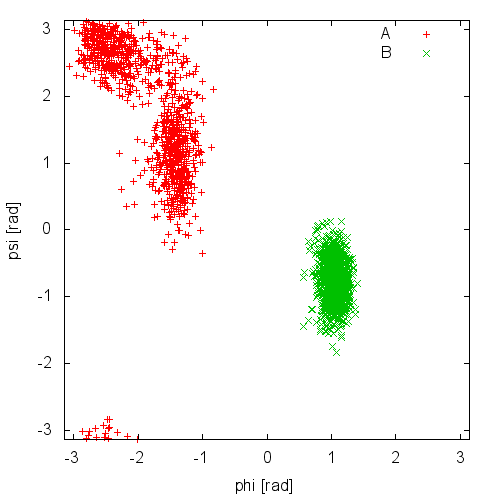(phi,psi) scatter plot obtained starting from two different structures. Simulation performed in vacuum.

Notice that the result depends heavily on the starting structure. For the simulation in vacuum, the two free-energy minima are separated by a large barrier and, in such a short simulation, the system cannot cross it. In water the barrier is smaller and you might see some crossing. Also notice that the two clouds are well separated, indicating that these two collective variables are good enough to properly distinguish among the two minima.

As a final comment, notice that if you run twice the same calculation in the same directory, you might overwrite the resulting files. GROMACS takes automatic backup of the output files, and PLUMED does it as well. In case you are restarting a simulation, you can add the keyword RESTART at the beginning of the PLUMED input file. This will tell PLUMED to append files instead of taking a backup copy.

# Biasing collective variables

We have seen that PLUMED can be used to compute collective variables. However, PLUMED is most often use to add forces on the collective variables. To this aim, we have implemented a variety of possible biases acting on collective variables. A bias works in a manner conceptually similar to the PRINT command, taking as argument one or more collective variables. However, here the STRIDE is usually omitted (that is equivalent to setting it to 1), which means that forces are applied at every timestep. In PLUMED 2.2 you will be able to change the STRIDE also for bias potentials, but that's another story. In the following we will see how to apply harmonic restraints and how to build an adaptive bias potential with metadynamics. The complete documentation for all the biasing methods available in PLUMED can be found at the Bias page.

If you do not know exactly where you would like your collective variables to go, and just know (or suspect) that some variables have large free-energy barriers that hinder some conformational rearrangement or some chemical reaction, you can bias them using metadynamics. In this way, a time dependent, adaptive potential will be constructed that tends to disfavor visited configurations in the collective-variable space. The bias is usually built as a sum of Gaussian deposited in the already visited states.

### Exercise 1

Now run a metadynamics simulation with the following input

phi: TORSION ATOMS=5,7,9,15
psi: TORSION ATOMS=7,9,15,17
METAD ARG=phi,psi HEIGHT=1.0 BIASFACTOR=10 SIGMA=0.35,0.35 PACE=100 GRID_MIN=-pi,-pi GRID_MAX=pi,pi


(see TORSION and METAD) Thus, a single METAD line will contain all the metadynamics related options, such as Gaussian height (HEIGHT, here in kJ/mol), stride (PACE, here in number of time steps), bias factor (BIASFACTOR, here indicates that we are going to effectively boost the temperature of the collective variables by a factor 10), and width (SIGMA, an array with same size as the number of collective variables).

There are two additional keywords that are optional, namely GRID_MIN and GRID_MAX. These keywords sets the range of the collective variables and tell PLUMED to keep the bias potential stored on a grid. This affects speed but, in principle, not the accuracy of the calculation. You can try to remove those keywords and see the difference.

Now, run a metadynamics simulations and check the explored collective variable space. Results from a 200ps (100000 steps) trajectory in vacuum are shown in Figure munster-ala-traj-metad.(phi,psi) scatter plot obtained with metadynamics and two different values of the biasfactor. Simulation performed in vacuum.

As you can see, exploration is greatly enhanced. Notice that the explored ensemble can be tuned using the biasfactor $$\gamma$$. Larger $$\gamma$$ implies that the system will explore states with higher free energy. As a rule of thumb, if you expect a barrier of the order of $$\Delta G^*$$, a reasonable choice for the biasfactor is $$\gamma\approx\frac{\Delta G}{2k_BT}$$.

Finally, notice that METAD potential depends on the previously visited trajectories. As such, when you restart a previous simulation, it should read the previously deposited HILLS file. This is automatically triggered by the RESTART keyword.

### Exercise 2

In this exercise, we will run a well-tempered metadynamics simulation on alanine dipeptide in vacuum, using as CV the backbone dihedral angle phi. In order to run this simulation we need to prepare the PLUMED input file (plumed.dat) as follows.

# set up two variables for Phi and Psi dihedral angles
phi: TORSION ATOMS=5,7,9,15
psi: TORSION ATOMS=7,9,15,17
#
# Activate well-tempered metadynamics in phi depositing
# a Gaussian every 500 time steps, with initial height equal
# to 1.2 kJoule/mol, biasfactor equal to 10.0, and width to 0.35 rad

ARG=phi
PACE=500
HEIGHT=1.2
SIGMA=0.35
FILE=HILLS
BIASFACTOR=10.0
TEMP=300.0
GRID_MIN=-pi
GRID_MAX=pi
GRID_SPACING=0.1

# monitor the two variables and the metadynamics bias potential
PRINT STRIDE=10 ARG=phi,psi,metad.bias FILE=COLVAR

The syntax for the command METAD is simple. The directive is followed by a keyword ARG followed by the labels of the CVs on which the metadynamics potential will act. The keyword PACE determines the stride of Gaussian deposition in number of time steps, while the keyword HEIGHT specifies the height of the Gaussian in kJoule/mol. For each CVs, one has to specified the width of the Gaussian by using the keyword SIGMA. Gaussian will be written to the file indicated by the keyword FILE.

The bias potential will be stored on a grid, whose boundaries are specified by the keywords GRID_MIN and GRID_MAX. Notice that you should provide either the number of bins for every collective variable (GRID_BIN) or the desired grid spacing (GRID_SPACING). In case you provide both PLUMED will use the most conservative choice (highest number of bins) for each dimension. In case you do not provide any information about bin size (neither GRID_BIN nor GRID_SPACING) and if Gaussian width is fixed PLUMED will use 1/5 of the Gaussian width as grid spacing. This default choice should be reasonable for most applications.

Once the PLUMED input file is prepared, one has to run Gromacs with the option to activate PLUMED and read the input file:

mdrun_mpi -s ../TOPO/topolA.tpr -plumed plumed.dat -nsteps 5000000


During the metadynamics simulation, PLUMED will create two files, named COLVAR and HILLS. The COLVAR file contains all the information specified by the PRINT command, in this case the value of the CVs every 10 steps of simulation, along with the current value of the metadynamics bias potential. We can use COLVAR to visualize the behavior of the CV during the simulation: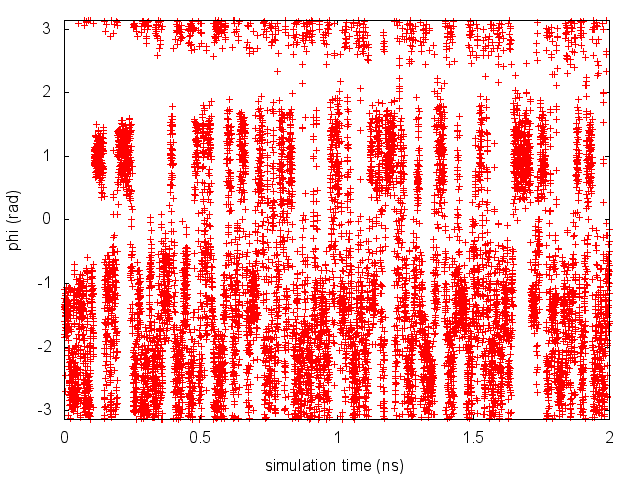Time evolution of the CV phi during the first 2 ns of a metadynamics simulation of alanine dipeptide in vacuum.

By inspecting Figure munster-metad-phi-fig, we can see that the system is initialized in one of the two metastable states of alanine dipeptide. After a while (t=0.1 ns), the system is pushed by the metadynamics bias potential to visit the other local minimum. As the simulation continues, the bias potential fills the underlying free-energy landscape, and the system is able to diffuse in the entire phase space.

The HILLS file contains a list of the Gaussians deposited along the simulation. If we give a look at the header of this file, we can find relevant information about its content:

#! FIELDS time phi psi sigma_phi sigma_psi height biasf
#! SET multivariate false
#! SET min_phi -pi
#! SET max_phi pi
#! SET min_psi -pi
#! SET max_psi pi


The line starting with FIELDS tells us what is displayed in the various columns of the HILLS file: the time of the simulation, the value of phi and psi, the width of the Gaussian in phi and psi, the height of the Gaussian, and the biasfactor. We can use the HILLS file to visualize the decrease of the Gaussian height during the simulation, according to the well-tempered recipe: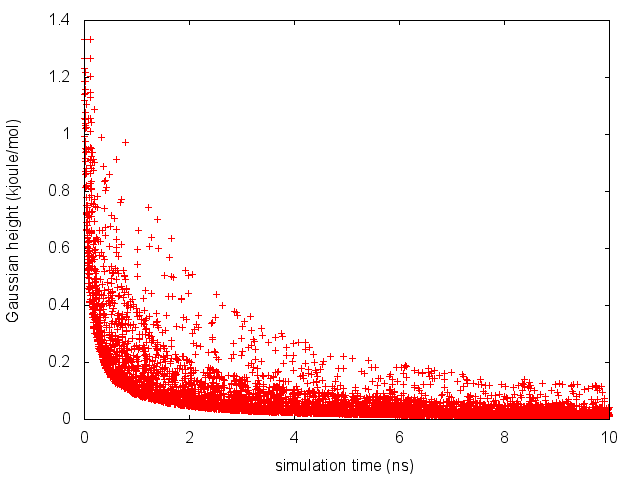Time evolution of the Gaussian height.

If we look carefully at the scale of the y-axis, we will notice that in the beginning the value of the Gaussian height is higher than the initial height specified in the input file, which should be 1.2 kJoule/mol. In fact, this column reports the height of the Gaussian rescaled by the pre-factor that in well-tempered metadynamics relates the bias potential to the free energy. In this way, when we will use sum_hills, the sum of the Gaussians deposited will directly provide the free-energy, without further rescaling needed (see below).

One can estimate the free energy as a function of the metadynamics CVs directly from the metadynamics bias potential. In order to do so, the utility sum_hills should be used to sum the Gaussians deposited during the simulation and stored in the HILLS file. To calculate the free energy as a function of phi, it is sufficient to use the following command line:

plumed sum_hills --hills HILLS


The command above generates a file called fes.dat in which the free-energy surface as function of phi is calculated on a regular grid. One can modify the default name for the free energy file, as well as the boundaries and bin size of the grid, by using the following options of sum_hills :

--outfile - specify the outputfile for sumhills
--min - the lower bounds for the grid
--max - the upper bounds for the grid
--bin - the number of bins for the grid
--spacing - grid spacing, alternative to the number of bins


The result should look like this:Estimate of the free energy as a function of the dihedral phi from a 10ns-long well-tempered metadynamics simulation.

To assess the convergence of a metadynamics simulation, one can calculate the estimate of the free energy as a function of simulation time. At convergence, the reconstructed profiles should be similar. The option –stride should be used to give an estimate of the free energy every N Gaussians deposited, and the option –mintozero can be used to align the profiles by setting the global minimum to zero. If we use the following command line:

plumed sum_hills --hills HILLS --stride 100 --mintozero


one free energy is calculated every 100 Gaussians deposited, and the global minimum is set to zero in all profiles. The resulting plot should look like the following: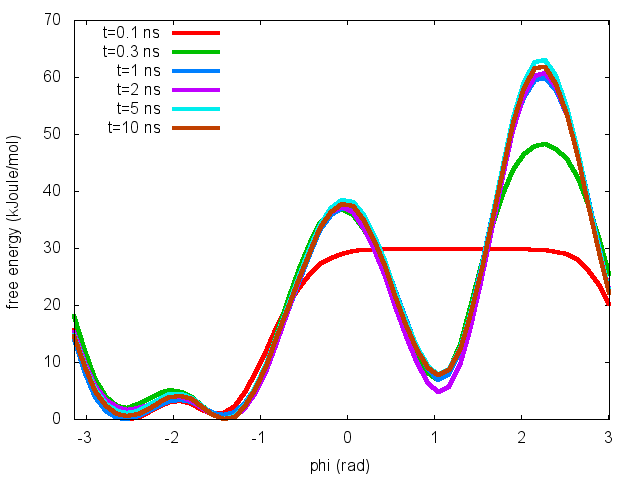Estimates of the free energy as a function of the dihedral phi calculated every 100 Gaussians deposited.

To assess the convergence of the simulation more quantitatively, we can calculate the free-energy difference between the two local minima of the free energy along phi as a function of simulation time. We can use following script to integrate the multiple free-energy profiles in the two basins defined by the following intervals in phi space: basin A, -3<phi<-1, basin B, 0.5<phi<1.5.

# number of free-energy profiles
nfes= # put here the number of profiles
# minimum of basin A
minA=-3
# maximum of basin A
maxA=1
# minimum of basin B
minB=0.5
# maximum of basin B
maxB=1.5
# temperature in energy units
kbt=2.5

for((i=0;i<nfes;i++))
do
# calculate free-energy of basin A
A=awk 'BEGIN{tot=0.0}{if($1!="#!" &&$1>min && $1<max)tot+=exp(-$2/kbt)}END{print -kbt*log(tot)}' min=${minA} max=${maxA} kbt=${kbt} fes_${i}.dat
# and basin B
B=awk 'BEGIN{tot=0.0}{if($1!="#!" &&$1>min && $1<max)tot+=exp(-$2/kbt)}END{print -kbt*log(tot)}' min=${minB} max=${maxB} kbt=${kbt} fes_${i}.dat
# calculate difference
Delta=$(echo "${A} - ${B}" | bc -l) # print it echo$i $Delta done notice that nfes should be set to the number of profiles (free-energy estimates at different times of the simulation) generated by the option –stride of sum_hills.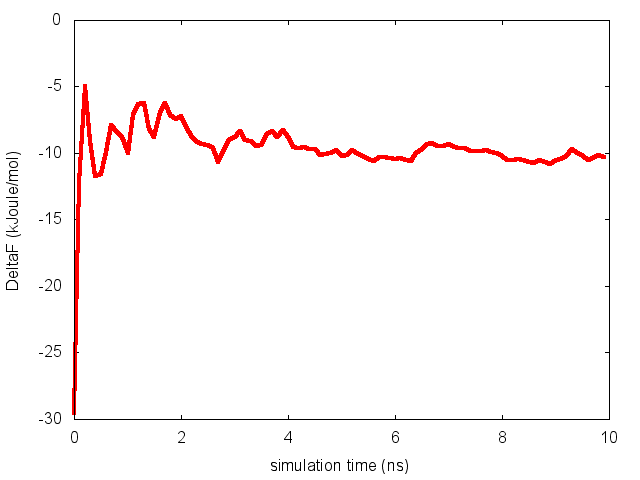Free-energy difference between basin A and B as a function of simulation time. This analysis, along with the observation of the diffusive behavior in the CVs space, suggest that the simulation is converged. ### Exercise 3 In this exercise, we will run a well-tempered metadynamics simulation on alanine dipeptide in vacuum, using as CV the backbone dihedral angle psi. In order to run this simulation we need to prepare the PLUMED input file (plumed.dat) as follows. # set up two variables for Phi and Psi dihedral angles phi: TORSION ATOMS=5,7,9,15 psi: TORSION ATOMS=7,9,15,17 # # Activate well-tempered metadynamics in psi depositing # a Gaussian every 500 time steps, with initial height equal # to 1.2 kJoule/mol, biasfactor equal to 10.0, and width to 0.35 rad METAD ... LABEL=metad ARG=psi PACE=500 HEIGHT=1.2 SIGMA=0.35 FILE=HILLS BIASFACTOR=10.0 TEMP=300.0 GRID_MIN=-pi GRID_MAX=pi GRID_SPACING=0.1 ... METAD # monitor the two variables and the metadynamics bias potential PRINT STRIDE=10 ARG=phi,psi,metad.bias FILE=COLVAR (see TORSION, METAD, and PRINT). Once the PLUMED input file is prepared, one has to run Gromacs with the option to activate PLUMED and read the input file: mdrun_mpi -s ../TOPO/topolA.tpr -plumed plumed.dat -nsteps 5000000  As we did in the previous exercise, we can use COLVAR to visualize the behavior of the CV during the simulation. Here we will plot at the same time the evolution of the metadynamics CV psi and of the other dihedral phi:Time evolution of the dihedrals phi and psi during a 10-ns long metadynamics simulation using psi as CV. By inspecting Figure munster-metad-psi-phi-fig, we notice that something different happened compared to the previous exercise. At first the behavior of psi looks diffusive in the entire CV space. However, around t=1 ns, psi seems trapped in a region of the CV space in which it was previously diffusing without problems. The reason is that the non-biased CV phi after a while has jumped into a different local minima. Since phi is not directly biased, one has to wait for this (slow) degree of freedom to equilibrate before the free energy along psi can converge. Try to repeat the analysis done in the previous exercise (calculate the estimate of the free energy as a function of time and monitor the free-energy difference between basins) to assess the convergence of this metadynamics simulation. ## Restraints To learn more: Biased sampling theory To learn more: Umbrella sampling theory If you want to just bring a collective variables to a specific value, you can use a simple restraint. Let's imagine that we want to force the $$\Phi$$ angle to visit a region close to $$\Phi=\pi/2$$. We can do it adding a restraint in $$\Phi$$, with the following input phi: TORSION ATOMS=5,7,9,15 psi: TORSION ATOMS=7,9,15,17 res: RESTRAINT ARG=phi AT=0.5pi KAPPA=5 PRINT ARG=phi,psi,res.bias  (see TORSION, RESTRAINT, and PRINT). Notice that here we are printing a quantity named res.bias. We do this because RESTRAINT does not define a single value (that here would be theoretically named res) but a structure with several components. All biasing methods (including METAD) do so, as well as many collective variables (see e.g. DISTANCE used with COMPONENTS keyword). Printing the bias allows one to know how much a given snapshop was penalized. Also notice that PLUMED understands numbers in the for {number}pi. This is convenient when using torsions, since they are expressed in radians. Now you can plot your trajectory with gnuplot and see the effect of KAPPA. You can also try different values of KAPPA. The stiffer the restraint, the less the collective variable will fluctuate. However, notice that a too large kappa could make the MD integrator unstable. To learn more: Moving restraints ## Using multiple replicas Warning Notice that multireplica simulations with PLUMED are fully supported with GROMACS, but only partly supported with other MD engines. Some free-energy methods are intrinsically parallel and requires running several simultaneous simulations. This can be done with gromacs using the multi replica framework. That is, if you have 4 tpr files named topol0.tpr, topol1.tpr, topol2.tpr, topol3.tpr you can run 4 simultaneous simulations. > mpirun -np 4 mdrun_mpi -s topol.tpr -plumed plumed.dat -multi 4 -nsteps 500000  Each of the 4 replicas will open a different topol file, and GROMACS will take care of adding the replica number before the .tpr suffix. PLUMED deals with the extra number in a slightly different way. In this case, for example, PLUMED first look for a file named plumed.dat.X, where X is the number of the replica. In case the file is not found, then PLUMED looks for plumed.dat. If also this is not found, PLUMED will complain. As a consequence, if all the replicas should use the same input file it is sufficient to put a single plumed.dat file, but one has also the flexibility of using separate files named plumed.dat.0, plumed.dat.1 etc. Finally, notice that the way PLUMED adds suffixes will change in version 2.2, and names will be plumed.0.dat etc. Also notice that providing the flag -replex one can instruct gromacs to perform a replica exchange simulation. Namely, from time to time gromacs will try to swap coordinates among neighboring replicas and accept of reject the exchange with a Monte Carlo procedure which also takes into account the bias potentials acting on the replicas, even if different bias potentials are used in different replicas. That is, PLUMED allows to easily implement many forms of Hamiltonian replica exchange. ## Using multiple restraints with replica exchange To learn more: Weighted histogram analysis method theory ### Exercise 4 In this exercise we will run multiple restraint simulations and learn how to reweight and combine data with WHAM to obtain free-energy profiles. We start with running in a replica-exchange scheme 32 simulations with a restraint on phi in different positions, ranging from -3 to 3. We will instruct gromacs to attempt an exchange between different simulations every 1000 steps. nrep=32 dx=echo "6.0 / ($nrep - 1 )" | bc -l

for((i=0;i<nrep;i++))
do
# center of the restraint
AT=echo "$i *$dx - 3.0" | bc -l

cat >plumed.dat.$i << EOF phi: TORSION ATOMS=5,7,9,15 psi: TORSION ATOMS=7,9,15,17 # # Impose an umbrella potential on phi # with a spring constant of 200 kjoule/mol # and centered in phi=AT # restraint-phi: RESTRAINT ARG=phi KAPPA=200.0 AT=$AT
# monitor the two variables and the bias potential
PRINT STRIDE=100 ARG=phi,psi,restraint-phi.bias FILE=COLVAR
EOF

# we initialize some replicas in A and some in B:
if((i%2==0)); then
cp ../TOPO/topolA.tpr topol$i.tpr else cp ../TOPO/topolB.tpr topol$i.tpr
fi
done

# run REM
mpirun -np $nrep mdrun_mpi -plumed plumed.dat -s topol.tpr -multi$nrep -replex 1000 -nsteps 500000


To be able to combine data from all the simulations, it is necessary to have an overlap between statistics collected in two adjacent umbrellas. Have a look at the plot of (phi,psi) for the different simulations to understand what is happening.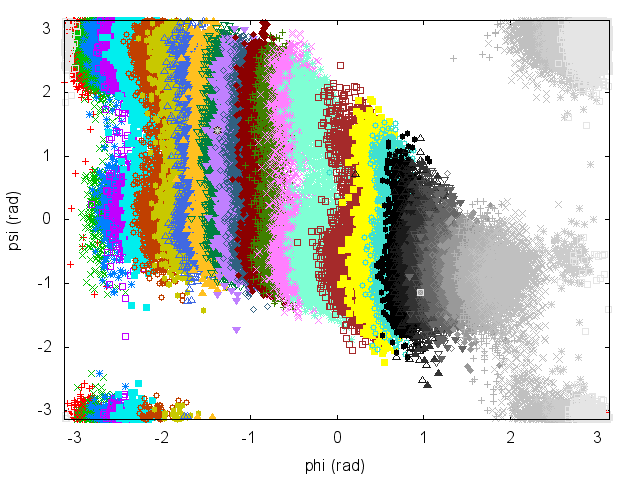(phi,psi) scatter plot from the multiple restraints simulation.

An often misunderstood fact about WHAM is that data of the different trajectories can be mixed and it is not necessary to keep track of which restraint was used to produce every single frame. Let's get the concatenated trajectory

trjcat_mpi -cat -f traj*.xtc -o alltraj.xtc


Now we should compute the value of each of the bias potentials on the entire (concatenated) trajectory.

nrep=32
dx=echo "6.0 / ( $nrep - 1 )" | bc -l for i in seq 0$(( $nrep - 1 )) do # center of the restraint AT=echo "$i * $dx - 3.0" | bc -l cat >plumed.dat << EOF phi: TORSION ATOMS=5,7,9,15 psi: TORSION ATOMS=7,9,15,17 restraint-phi: RESTRAINT ARG=phi KAPPA=200.0 AT=$AT

# monitor the two variables and the bias potential
PRINT STRIDE=100 ARG=phi,psi,restraint-phi.bias FILE=ALLCOLVAR.$i EOF plumed driver --mf_xtc alltraj.xtc --trajectory-stride=10 --plumed plumed.dat done  It is very important that this script is consistent with the one used to generate the multiple simulations above. Now, single files named ALLCOLVAR.XX will contain on the fourth column the value of the bias centered in a given position, computed on the entire concatenated trajectory. Next step is to compute the weights self-consistently solving the WHAM equations, using the python script "wham.py" contained in the SCRIPTS directory. To use this code: ../SCRIPTS/wham.sh ALLCOLVAR.*  This script will produce several files. Let's visualize "phi_fes.dat", which contains the free energy as a function of phi, and compare this with the result previously obtained with metadynamics.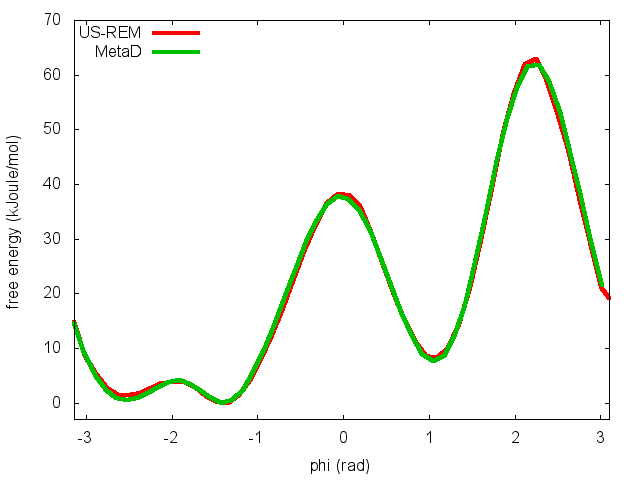Free energy as a function of phi from multiple restraint (US-REM) and metadynamics (MetaD) simulations. ### Exercise 5 In the previous exercise, we use multiple restraint simulations to calculate the free energy as a function of the dihedral phi. The resulting free energy was in excellent agreement with our previous metadynamics simulation. In this exercise we will repeat the same procedure for the dihedral psi. At the end of the steps defined above, we can plot the free energy "psi_fes.dat" and compare it with the reference profile calculated from a metadynamics simulations using both phi and psi as CVs.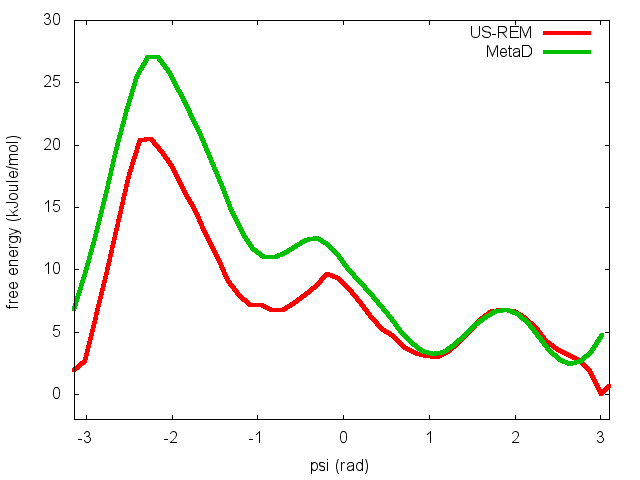Free energy as a function of psi from multiple restraint (US-REM) and metadynamics (MetaD) simulations. We can easily spot from the plot above that something went wrong in this multiple restraint simulations, despite we used the very same approach we adopted for the phi dihedral. The problem here is that psi is a "bad" collective variable, and the system is not able to equilibrate the missing slow degree of freedom phi in the short time scale of the umbrella simulation (1 ns). In the metadynamics exercise in which we biased only psi, we detect problems by observing the behavior of the CV as a function of simulation time. How can we detect problems in multiple restraint simulations? This is slightly more complicated, but running this kind of simulation in a replica-exchange scheme offers a convenient way to detect problems. The first thing we need to do is to demux the replica-exchange trajectories and reconstruct the continous trajectories of the replicas across the different restraint potentials. In order to do so, we can use the following script: demux.pl md0.log trjcat_mpi -f traj*.xtc -demux replica_index.xvg  This commands will generate 32 continous trajectories, named XX_trajout.xtc. We will use the driver to calculate the value of the CVs phi and psi on these trajectories. nrep=32 for i in seq 0$(( $nrep - 1 )) do cat >plumed.dat << EOF phi: TORSION ATOMS=5,7,9,15 psi: TORSION ATOMS=7,9,15,17 # monitor the two variables PRINT STRIDE=100 ARG=phi,psi FILE=COLVARDEMUX.$i
EOF

plumed driver --mf_xtc \${i}_trajout.xtc --trajectory-stride=10 --plumed plumed.dat

done


The COLVARDEMUX.XX files will contain the value of the CVs on the demuxed trajectory. If we visualize these files we will notice that replicas sample the CVs space differently. In order for each umbrella to equilibrate the slow degrees of freedom phi, the continuous replicas must be ergodic and thus sample the same distribution in phi and psi.(phi,psi) scatter plot from the demuxed trajectories of the multiple restraints simulation in psi.